## Example Questions

### Example Question #11 : How To Find Percentage

What number is 30% of 90?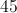Explanation:

Verbal cues include "is" and "of."  "Is" means equals and "of" means multiplication.

So the equation to solve becomes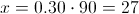### Example Question #11 : How To Find Percentage

If the length of a rectangle is increased by 50% and with width of the same rectangle is decreased by 50%, what is the effect on the area of the rectangle?

It is unchanged

It is increased by 50%

It is increased by 25%

It is decreased by 50%

It is decreaed by 25%

It is decreaed by 25%

Explanation:

This is a good question to use random numbers. Lets use 100 and 10 as the lengths. That means the area = 100 x 10 = 1000

If we increase the length of the side measuring 100 by 50% = 150

If we decrease the length of the other side 50% = 5

Area = 150 x 5 = 750

We see that the area decreased by 250 or 25% decrease in area!

### Example Question #13 : How To Find Percentage

If 80 percent of x is 200, what is 60 percent x?

175

200

150

250

225

150

Explanation: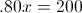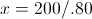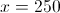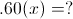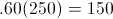### Example Question #14 : How To Find Percentage

Seventeen percent of some number is 34. What is this number?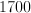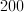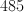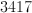Explanation:

The algebraic expression is: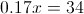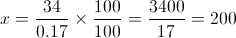### Example Question #12 : How To Find Percentage

What number is 125% of 60?

75

80

66

90

60

75

Explanation:

Translate this into a mathematical equation:

x = 1.25 * 60

x = 75

### Example Question #13 : How To Find Percentage

If 10% ofis equal to 25% of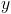, and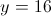, what is the value of?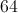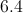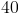Explanation:

Find 25% of: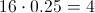10% ofis equal to 4. Therefore,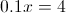.

Divide both sides by 0.1 to find that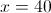.

### Example Question #11 : Fractions

There areregistered students in the math club. Students are deciding what activity they could do for their end of semester event. They have three choices: rock climbing, bowling, or outdoor laser tag.students vote for rock climbing,students vote for bowling, andstudents vote for outdoor laser tag.

What percent of students did not vote?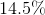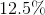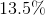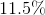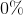Explanation:

The number of students who did not vote is: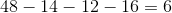The percent of students who did not vote is therefore: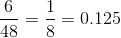of the students did not vote.

### Example Question #1161 : Sat Mathematics

Joaquin is running for Prom King.  If he receivesof the votes from a senior class ofstudents, and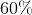of the votes from a junior class of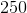students, how many votes did he receive in total?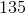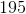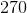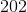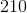Explanation:

First, we find how many seniors voted for him:

0.30 * 200 = 60 seniors

Then we find how many juniors using the same method

0.60 * 250 = 150 Juniors

Finally, we add the two together

60 + 150 = 210 students total

### Example Question #11 : Other Percentage

13 is what percent of 25?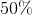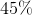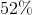Explanation:

To solve this problem, we should set up the following proportion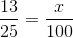where x is the percentage we are looking for.

To solve, simply cross multiply and solve for x.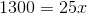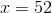### Example Question #13 : Other Percentage

What percent ofis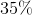Explanation:

Let's set up a proportion to solve this problem.

We're looking for a percentage,.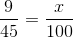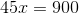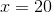Our answer is### All SAT Math Resources